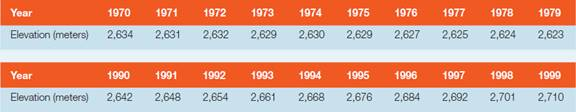Chapter 16, Problem 1PA

Chapter
Section
Textbook Problem

The table shows the position (elevation) of the toe of a Northern Hemisphere valley glacier as measured yearly on September 30 during the 1970s, then again during the 1990s. Use decadal averages and annual rates of change of position to help you describe how the behavior of the glacier in the 1990s differs from that of the 1970s.To determine

The way in which behavior of the glacier in the 1990s differs from that of the 1970s using the decadal averages and annual rates of change of position of the toe of a Northern Hemisphere valley glacier as measured, yearly on September 30 during the 1970s, then again during the 1990s.

Explanation

To find the average elevation in the 1970s and 1990s, the annual rates of change in elevation need to be added and divided by the number of years. It is expressed as follows:

Average elevation (in meters) =  The sum of annual rates of change of position of toeTotal number of years

Therefore:

Average elevation for 1970s =  26,28410=2628.4 meters

Average elevation for 1990s =  26,73610=2673.6 meters

Hence, the average elevation in the 1970s was 2628.4 meters and in the 1990s, it was 2673.6 meters.

A lower elevation indicates that the glacier is growing and advancing. Hence, in the 1970s, the glacier was undergoing net accumulation and advancing down the valley and in the 1990s. It was experiencing net ablation and retreating up the valley.

To find the average annual rate of change in the 1970s,

First, subtract the elevation of the glacier's toe in one year from its position in the next year. Then, average those annual values by dividing with the total number of pairs of years, which is nine.

The general formula to find the annual rate of change for a pair of year is expressed as follows:

Annual rate of change = (The elevation of glacier's toe on a year)(The elevation of glacier's toe on a preceding year)

For example, subtract the elevation of glacier’s toe on the year 1971 with 1970 from the given table, likewise for all the nine pairs.

Therefore:

Annual rate of change for 1970 and 1971 = 26312634=3

Still sussing out bartleby?

Check out a sample textbook solution.

See a sample solution

The Solution to Your Study Problems

Bartleby provides explanations to thousands of textbook problems written by our experts, many with advanced degrees!

Get Started

Name the following molecular compounds a ClF4 b CS2 c PF3 d SF6

General Chemistry - Standalone book (MindTap Course List)

How do eating disorders affect health?

Understanding Nutrition (MindTap Course List)

What evidence shows that white dwarfs must be very small?

Horizons: Exploring the Universe (MindTap Course List)

What are sister chromatids?

Human Heredity: Principles and Issues (MindTap Course List)